1.CPU 推理性能提升 2-4 倍，模型大小降低至1/4，模型量化真的这么好使？

• 减少内存带宽和存储空间

• 提高系统吞吐量（throughput），降低系统延时（latency）

2.量化设计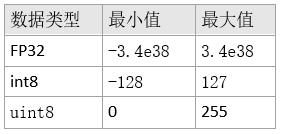• 非对称算法 （asymmetric）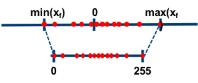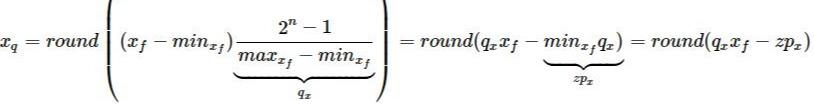• 对称算法（symmetric）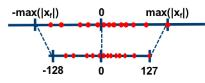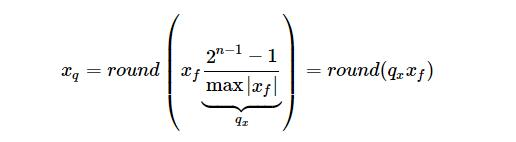• 对称算法 VS 非对称的算法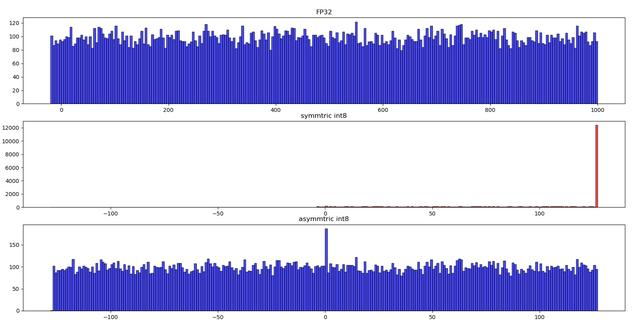• 引入 zero-point 对算子的影响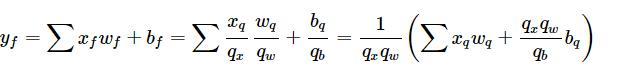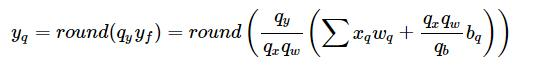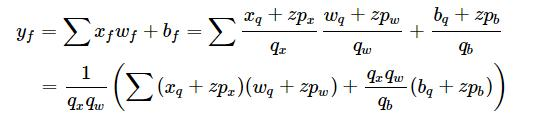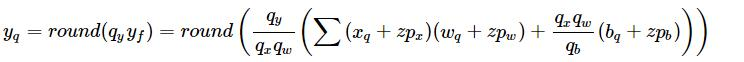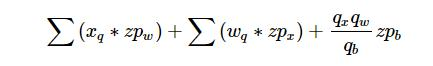• 浮点数动态范围的选取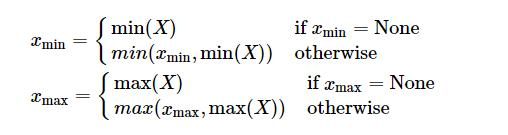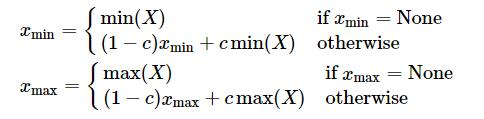KL 距离采样方法(Kullback–Leibler divergence)【8】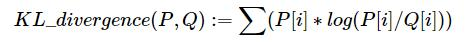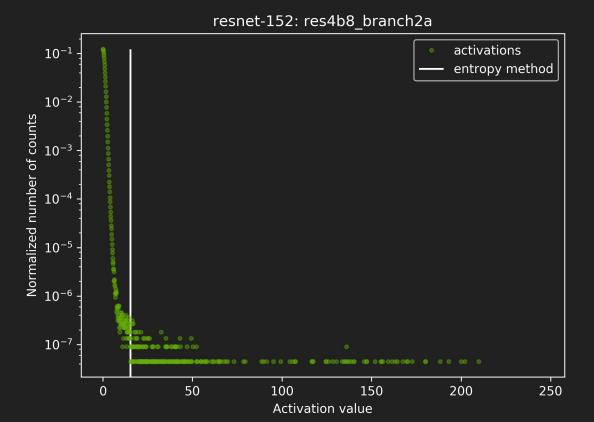KL 距离采样方法通过直方图来模拟两个分布。其伪代码如下：

``````1 Input: FP32 histogram H with 2048 bins: bin[ 0 ], …, bin[ 2047 ]

2 For i in range( 128 , 2048 ):

3 reference_distribution_P = [ bin[ 0 ] , ..., bin[ i-1 ] ] // take first ‘ i ‘ bins from H

4 outliers_count = sum( bin[ i ] , bin[ i+1 ] , … , bin[ 2047 ] )

5 reference_distribution_P[ i-1 ] += outliers_count

6 P /= sum(P) // normalize distribution P

7 candidate_distribution_Q = quantize [ bin[ 0 ], …, bin[ i-1 ] ] into 128 levels // explained later

8 expand candidate_distribution_Q to ‘ i ’ bins // explained later

9 Q /= sum(Q) // normalize distribution Q

10 divergence[ i ] = KL_divergence( reference_distribution_P, candidate_distribution_Q)

11 End For

12 Find index ‘m’ for which divergence[ m ] is minimal

13 threshold = ( m + 0.5 ) * ( width of a bin )``````

P = [ 1, 0, 2, 3, 5, 3, 1, 7] =》[1 + 0 + 2 + 3 , 5 + 3 + 1 + 7] = [6, 16]

Q = [ 6/3, 0, 6/3, 6/3, 16/4, 16/4, 16/4, 16/4] = [ 2, 0, 2, 2, 4, 4, 4, 4]

• 量化粒度

Activation 和 weights 都可以看做是一个张量，因此在这种量化方式，两者并没有区别。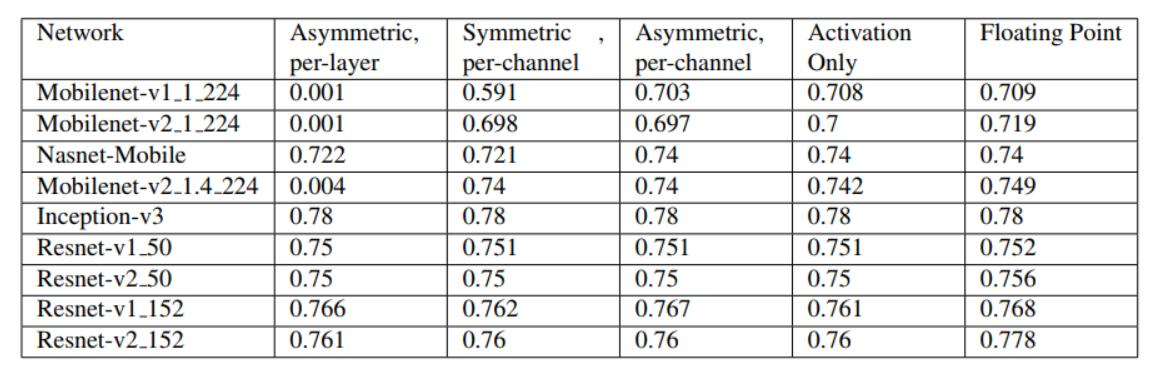• Calibration

3. Pytorch 模型量化实战

• 不得不谈的 torch.nn.Module 类

torch.nn.Module 类是所有神经网络模型的基类。一个模块可以包含子模块，通常一个神经网络模型也是一个模块，它以树状结构来组织各个子模块。比如，一个 resnet50 模型是一个模块，一个torch.nn.Conv2d 算子也可以封装成一个模块。下面的一个例子展示了一个含有两个卷积操作的简单模型。

``````import torch.nn as nn

import torch.nn.functional as F

class Model(nn.Module):

def __init__(self):

super(Model, self).__init__()

self.conv1 = nn.Conv2d(1, 20, 5)

self.conv2 = nn.Conv2d(20, 20, 5)

def forward(self, x):

x = F.relu(self.conv1(x))

return F.relu(self.conv2(x))``````

Model 本身也是 Module 类的子类，Module 类的子类都需要定义 __init_ 和 forward，前者定义子类的树状结构，后者定义计算逻辑。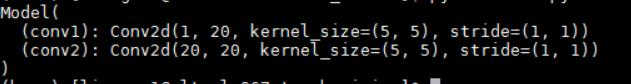• Pytorch Post-training 量化的基本步骤【9】

1）准备模型：

2）fuse modules

3）设置量化算法

4）传播 qconfig 和插入 observer

5） calibration

6）module 转化

• Pytorch 量化 resnet50

``````class Bottleneck(nn.Module):

expansion = 4

__constants__ = ['downsample']

def __init__(self, inplanes, planes, stride=1, downsample=None, groups=1,

base_width=64, dilation=1, norm_layer=None):

super(Bottleneck, self).__init__()

if norm_layer is None:

norm_layer = nn.BatchNorm2d

width = int(planes * (base_width / 64.)) * groups

# Both self.conv2 and self.downsample layers downsample the input when stride != 1

self.conv1 = conv1x1(inplanes, width)

self.bn1 = norm_layer(width)

self.conv2 = conv3x3(width, width, stride, groups, dilation)

self.bn2 = norm_layer(width)

self.conv3 = conv1x1(width, planes * self.expansion)

self.bn3 = norm_layer(planes * self.expansion)

self.relu = nn.ReLU(inplace=True)

self.downsample = downsample

self.stride = stride

def forward(self, x):

identity = x

out = self.conv1(x)

out = self.bn1(out)

out = self.relu(out)

out = self.conv2(out)

out = self.bn2(out)

out = self.relu(out)

out = self.conv3(out)

out = self.bn3(out)

if self.downsample is not None:

identity = self.downsample(x)

out += identity

out = self.relu(out)

return out

class QuantizableBottleneck(Bottleneck):

def __init__(self, *args, **kwargs):

super(QuantizableBottleneck, self).__init__(*args, **kwargs)

self.relu1 = nn.ReLU(inplace=False)

self.relu2 = nn.ReLU(inplace=False)

def forward(self, x):

identity = x

out = self.conv1(x)

out = self.bn1(out)

out = self.relu1(out)

out = self.conv2(out)

out = self.bn2(out)

out = self.relu2(out)

out = self.conv3(out)

out = self.bn3(out)

if self.downsample is not None:

identity = self.downsample(x)

return out

def fuse_model(self):

fuse_modules(self, [['conv1', 'bn1', 'relu1'],

['conv2', 'bn2', 'relu2'],

['conv3', 'bn3']], inplace=True)

if self.downsample:

torch.quantization.fuse_modules(self.downsample, ['0', '1'], inplace=True)

class QuantizableResNet(ResNet):

def __init__(self, *args, **kwargs):

super(QuantizableResNet, self).__init__(*args, **kwargs)

self.quant = torch.quantization.QuantStub()

self.dequant = torch.quantization.DeQuantStub()

def forward(self, x):

x = self.quant(x)

# Ensure scriptability

# super(QuantizableResNet,self).forward(x)

# is not scriptable

x = self._forward_impl(x)

x = self.dequant(x)

return x

def fuse_model(self):

r"""Fuse conv/bn/relu modules in resnet models

Fuse conv+bn+relu/ Conv+relu/conv+Bn modules to prepare for quantization.

Model is modified in place. Note that this operation does not change numerics

and the model after modification is in floating point

"""

fuse_modules(self, ['conv1', 'bn1', 'relu'], inplace=True)

for m in self.modules():

if type(m) == QuantizableBottleneck or type(m) == QuantizableBasicBlock:

m.fuse_model()

Calibration 和模型转换

if args.post_training_quantize:

# perform calibration on a subset of the training dataset

# for that, create a subset of the training dataset

ds = torch.utils.data.Subset(

dataset,

indices=list(range(args.batch_size * args.num_calibration_batches)))

ds, batch_size=args.batch_size, shuffle=False, num_workers=args.workers,

pin_memory=True)

model.eval()

model.fuse_model()

torch.save(model.state_dict(), "fp32_resnet_50.modle")

model.qconfig = torch.quantization.get_default_qconfig(args.backend)

torch.quantization.prepare(model, inplace=True)

# Calibrate first

print("Calibrating")

torch.quantization.convert(model, inplace=True)

if args.output_dir:

print('Saving quantized model')

if utils.is_main_process():

torch.save(model.state_dict(), os.path.join(args.output_dir,

'quantized_post_train_model.pth'))

print("Evaluating post-training quantized model")

torch.save(model.state_dict(), "int8_resnet_50.modle")

return``````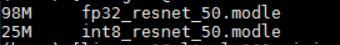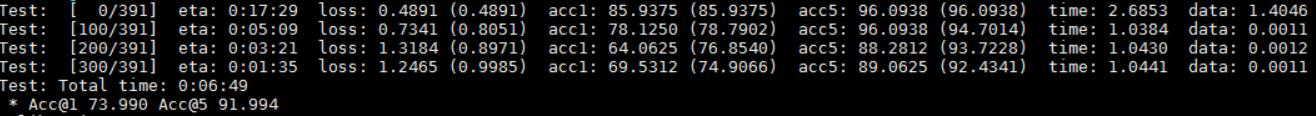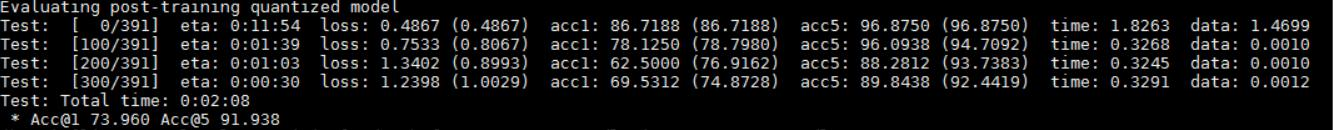git clone https://github.com/pytorch/vision.git

cd vision

python references/classification/train_quantization.py --data-path='xxx/imagenet/img/' --device='cpu' --test-only --backend='fbgemm' --model='resnet50' --post-training-quantize

4.经验分享

• 精度问题

• 性能问题

• sky-lake CPU 溢出问题

5.目前有哪些针对低精度推理的工具包？

• 深度学习框架

• 加速包
1. MKLDNN/MKL
2. FBGEMM
3. TensorRT
4. QNNPACK

6.总结

7.参考文献

1.Quantization wikipedia

https://en.wikipedia.org/wiki/Quantization_(signal_processing)

2.低数值精度深度学习推理与训练

Lower Numerical Precision Deep Learning Inference and Training

3.Quantization Algorithms

https://nervanasystems.github.io/distiller/algo_quantization.html

4.Quantizing deep convolutional networks for efficient inference: A whitepaper

https://arxiv.org/abs/1806.08342

5.Pytorch observer 实现

https://github.com/pytorch/pytorch/blob/master/torch/quantization/observer.py

6.GMMLOMP

7.FBGEMM

https://engineering.fb.com/ml-applications/fbgemm/

https://github.com/pytorch/FBGEMM

8.8-bit Inference with TensorRT

http://on-demand.gputechconf.com/gtc/2017/presentation/s7310-8-bit-inference-with-tensorrt.pdf

9.Pytorch Quantization Introduction

https://pytorch.org/docs/stable/quantization.html#quantization-workflows

10.性能问题

https://github.com/pytorch/pytorch/pull/30153

11.Pytorch tutorial

https://pytorch.org/tutorials/

12.Torchvision

https://github.com/pytorch/vision/tree/master/torchvision/models

13.Introduction to quantization

https://pytorch.org/docs/stable/quantization.html

14.What Is int8 Quantization and Why Is It Popular for Deep Neural Networks?

15.sky-lake 溢出问题

https://github.com/pytorch/FBGEMM/issues/125

### JavaScript 整分或者指定时间执行操作_js在指定时间执行-程序员宅基地

JavaScript 整分或者指定时间执行操作_js在指定时间执行

### python 头条_python-selenium登陆今日头条-程序员宅基地

https://blog.csdn.net/a942242856/article/details/88379727#python-selenium登陆今日头条在运营今日头条的过程当中，有时候未免要进行一些重复无味的劳动。比如在发放微头条的时候，写好了许多内容，并不像每次登陆然后逐个发表。比如我想每个整点去发表一些东西。那么自动登陆今日头条就很有必要了。选择selenium选择这个工具的原因是，它可..._selenium 今日头条

### 台式电脑怎么添加计算机硬盘,台式机如何添加硬盘扩大存储空间|如何对新添加的硬盘进行分区..._看你真有意思的博客-程序员宅基地

‍如果是家庭使用的电脑，很多人会选择台式机，在使用的过程中随着存储量不断增加，电脑系统里可用空间也越来越少，我们在不想让原有硬盘提前下岗的情况下，就可以在电脑上添加一个硬盘来扩大系统的磁盘容量。下面介绍如何在台式机上添加硬盘扩大存储空间。1、打开电脑机箱，用螺丝把新硬盘固定到硬盘槽里;2、给新装的硬盘插上电源插头，用sata串口硬盘线把硬盘的sata接口和主板上的sata接口连接;3、启动电脑，新..._给台式机加硬盘

### opencv+C++ 逐个遍历图像像素并进行修改_opencv修改像素点cpp-程序员宅基地

#include &quot;stdafx.h&quot;#include &quot;cv.h&quot;#include &quot;highgui.h&quot;#include &quot;cxcore.h&quot;#include &amp;lt;iostream&amp;gt;using namespace cv;using namespace std;#if 1int main(){ _opencv修改像素点cpp

### 惠普打印机故障代码_惠普打印机常见故障解决办法【图文详解】-程序员宅基地

Hp打印机，也就是我们常说的惠普打印机，目前已经成了打印机行业中的最为人们熟知的品牌之一，不过对于大多数打印机来说，无论品牌好坏，总会在使用的过程中出现这样那样的问题，而惠普打印机在使用过程中是故障率也是居高不下，如此看来掌握一定的维修技巧就显得十分必要了。今天小编就为大家总结几种惠普打印机常见的故障解决办法：1、打印时显示遇到DOT400X端口错误这种情况大多是由于USB端口出现了问题，要么是没..._惠普打印机故障编码